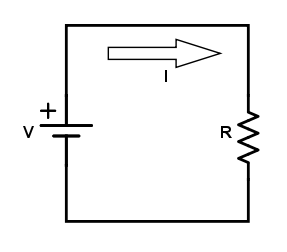# Ohm’s Law Calculator

## Calculate circuit parameters using Ohm's Law

Enter any two known values and press "Calculate" to solve for the others.
(V)
(A)
(‎Ω)
(W)

### Overview

When we talk about fundamental circuit theories, Ohm's Law is the most fundamental of them all. This law, which was presented by Georg Ohm in 1827, is probably the formula that all electrical and electronics engineers first learn. Nevertheless, we have designed this tool to help you compute for either the voltage, resistance or current if any other two parameters are given. To use this tool, just enter values to any two fields and press "calculate".### Equations

$$V = IR$$

$$P = VI$$

Where:

$$V$$ = voltage

$$I$$ = current

$$R$$ = resistance

$$P$$ = power

### Applications

The power dissipated by an electrical element can be expressed in terms of the voltage (or potential) across it and the current through it. In most cases, this power is converted to heat. According to Ohm's Law, power can also be calculated using $$P = I^{2}R$$ or $$P = \frac{V^{2}}{R}$$. This is why electrical power transmission lines use higher voltages because it will result in less power loss due to heating.

Another application is found in a common kitchen appliance: the toaster. Toasters heat bread by passing current to a nickel-chrome wire, which has high resistivity. Given the same current, materials with high resistivity theat up faster than ones with lower resistivity.  The same principle applies to incadescent bulbs, which converts electrical power to heat which makes the filament glow.